# DateTime in C#

##### How to use dates and times in c#?

Dates and Times:

It is all about representation of Date and Time, Timespan, DateTime, represents the dates and times in c#.

### Timespan:

A timespan represnts an interval of time, or time of the day, A timespan has a resolution of 100ns, Timespan has support a maximum value of 10 million days which may be positive or negative.

#### Timespan using constructor:

Timespan constructor is used to create new timespan.

#### Example program on Timespan constructor:

```using System;
using System.Collections.Generic;
using System.Linq;
using System.Text;
class Program
{
static void Main()
{

TimeSpan span = new TimeSpan(3, 2, 10, 30, 25);
Console.WriteLine(span);
}
}
```

In the above code snippet we have declared the timespan constructor. we have defined class as program and in the main function we have declared Timespan span as new Timespan (3, 2, 10, 30, 25). The values represent the day , hour, minutes, seconds, and milliseconds, In the next line we have defined console.writeline as span which gives the output as 3 days 2 hours, 10 minutes, 30 seconds and 25 milli seconds.

output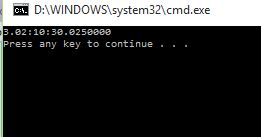Timespan using FromMethod:

FromMethod contains different methods called as Fromdays, FromHours, FromMinutes, FromSeconds.

#### Example program using FromMethod

```using System;
using System.Collections.Generic;
using System.Linq;
using System.Text;
class Program
{
static void Main()
{
TimeSpan span1 = TimeSpan.FromDays(20);
TimeSpan span2 = TimeSpan.FromHours(11);
TimeSpan span3 = TimeSpan.FromMinutes(46);
TimeSpan span4 = TimeSpan.FromSeconds(58);
TimeSpan span5 = TimeSpan.FromMilliseconds(59);

Console.WriteLine(span1);
Console.WriteLine(span2);
Console.WriteLine(span3);
Console.WriteLine(span4);
Console.WriteLine(span5);
}
}
```

In the above code snippet we have defined Timespan FromMethod. we have taken class as program and in the main function we have declared Timespan span1 to new Timespan.FromDays(20), Like that we have declared up to span5 and we have given console.writeline as span1 up to so on span5 which gives output values as days, Hours, minutes, seconds and milli seconds.

output: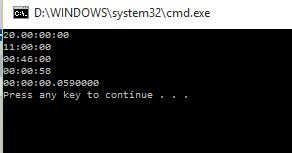```using System;
using System.Collections.Generic;
using System.Linq;
using System.Text;

class Program
{
static void Main()
{

TimeSpan span1 = TimeSpan.FromHours(6);
TimeSpan span2 = TimeSpan.FromMinutes(34);
Console.WriteLine(span3);
}
}
```

In the above code snippet we have defined Timespan AddMethod. In this program we have taken class as program  and in the main function we have declared Timespan span1 as Timespan.FromHours(6), In the next line we have taken Timespan span2 as Timespan.FromMinutes(34), In the third line we have given Timespan span3 = span1.ADD(span2) which adds the span2 value to span1 and in the next line we have given console.writeline as (span3) which gives the output as 06:34:00

output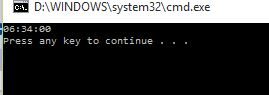#### Timespan as Subtract.Method:

Subtract.Method is used to subtract the values.

Example program on Timespan as subtract.Method

```using System;
using System.Collections.Generic;
using System.Linq;
using System.Text;
class Program
{
static void Main()
{

TimeSpan span1 = TimeSpan.FromMinutes(2);
TimeSpan span2 = TimeSpan.FromSeconds(1);
TimeSpan span3 = span1.Subtract(span2);
Console.WriteLine(span3);
}
}
```

In the above code snippet we have declared Timespan as subtract method. In this program we have taken class as program and in the main function we have taken Timespan span1 as Timespan.FromMinutes(2) and in the nextline we have taken Timespan span2 as Timespan .FromSeconds(1), In the nextline we have given the formula for subtracting the Timespan as Timespan span3 as span1.subtract(span2) here we are subtracting span2 as 1 second from span1 2 minutes and in the nextline we have given console.writeline which gives the output as 01:59.

output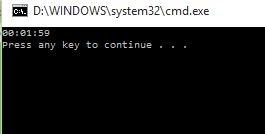### DateTime:

DateTime is used to represent a date and optionally a time they have a resolution of 100 nano seconds.

DateTime format:

For the correct formatting the datetime we use format.

#### Example program on DateTime Format:

```using System;
using System.Collections.Generic;
using System.Linq;
using System.Text;
class Program
{
static void Main()
{
DateTime time = DateTime.Now;
string format = "MMMM dddd d HH:mm yyyy";
Console.WriteLine(time.ToString(format));
}
}
```

In the above code snippet we have declared DateTimeformat. In this program we have taken class as program and in the main function we have taken datetime time as Datetime.now which represent present datetime and year and in the nextline we are writing string format as (MMMM dddd d HH:MM YYYY) which is used to display the complete details as month, day, date, Hours, Minutes and year and in the nextline we are giving the console.writeline as (time.Tostring (format)) here we are using ToString which is used to convert the DateTime to  current DateTime, which prints the output as current datetime.

```MMM     display three-letter month
ddd     display three-letter day of the WEEK
d       display day of the MONTH
HH      display two-digit hours on 24-hour
mm      display two-digit minutes
yyyy    display four-digit year```

output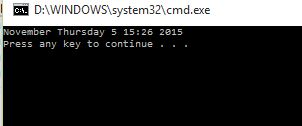#### Example program on Tostring method:

```using System;
using System.Collections.Generic;
using System.Linq;
using System.Text;
class Program
{
static void Main()
{
DateTime now = DateTime.Now;
Console.WriteLine(now.ToLongDateString());
Console.WriteLine(now.ToLongTimeString());
Console.WriteLine(now.ToShortDateString());
Console.WriteLine(now.ToShortTimeString());
Console.WriteLine(now.ToString());
}
}
```

In the above code snippet we have declared ToStringmethod. In this program we are finding current date time by using longDateTime and short DateTime. The long date and time displays the datetime in detail and short datetime displays the date time in short form, We have taken class as program. In the main function we have declared  datetime as now and we are giving console.writeline as (now.Tolongdatestring) which displays in detail, In the next line we are giving the console.writeline as (now.ToLongTimestring) which displays the time in detail and in the nextline we are giving the console.writeline as (now.To short date string) which displays the date in short form, In the next line we have given the console.writeline as (now.Toshorttime) which displays the time in short form and in the nextline we have given console.writeline as (now.Tostring) which is used to display the current datetime.

output#### Example program to find out days using date time in c#:

```using System;
using System.Collections.Generic;
using System.Linq;
using System.Text;
class Program
{
static void Main()
{
DateTime now = DateTime.Today;
for (int i = 0; i < 7; i++)
{
Console.WriteLine(now.ToString("dddd"));
}
}
}
```

In the above code snippet we are finding the days using datetime. In the above program we have taken class as program and in the main function we have taken (datetime now =datetime.Today) which takes the current date and time and we are using for condition to check the days we are using condition as (int i=0; i<7; i++) as zero starts from today and i<7 counts the 7 days from zero to seven and i++ is used to increment the day if it is less than the 7 and in the next line we are giving the console.writeline(now.ToString("dddd") which displays the current datetime with four digit day and in the last line we have given now =now.ADDDays(1) which prints the day by one value.

output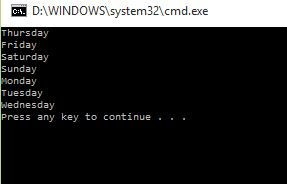#### Example program to find out AM and PM using DateTime:

```using System;
using System.Collections.Generic;
using System.Linq;
using System.Text;
class Program
{
static void Main()
{
DateTime now = DateTime.Now;
for (int i = 0; i < 2; i++)
{
Console.WriteLine(now.ToString("tt "));
}
}
}
```

In the above code snippet we are finding AM and PM using DateTime  we have taken class as program and in the main function we have taken datetime now=datetime.now which represents the current datetime and we are taking for condition int( i=0, i<2, i++) which represents the value i=0 and if the value is less than 2 it increments the value by the condition i++ and it increments by the value 1 and in the console.writeline condition we have given now.ToString("tt") which shoiws current time with two digits value and in the last line we have taken now=now.AddHours(12) which is used to increments by 12 shows as PM and AM after twelve hours.

outputViews: 6402 | Post Order: 17